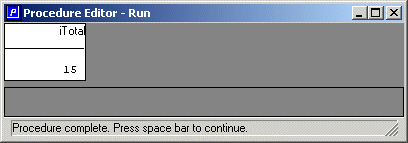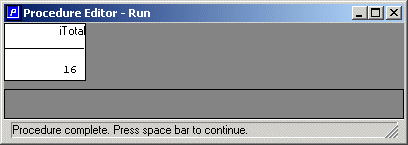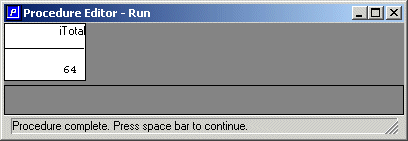ABL Essentials
Procedure Blocks and Data Access : Language statements that define blocks : DO blocks : Looping with a DO block

Looping with a DO block
To loop through a group of statements a specific number of times, use this form of the DO statement:
 DO variable = expression1 TO expression2[ BY constant]:
The following example adds up the integers from one through five and displays the total:
 DEFINE VARIABLE iCount AS INTEGER    NO-UNDO.DEFINE VARIABLE iTotal AS INTEGER    NO-UNDO.DO iCount = 1 TO 5:  iTotal = iTotal + iCount.END.DISPLAY iTotal.
The following figure shows the result.
Figure 6. Result of looping with a DO blockThe starting and ending values can be expressions and not just constants. You can use some value other than one to increment the starting value each time through the loop by using the BY phrase at the end. If the start and end values are variables or other expressions, ABL Virtual Machine (AVM) evaluates them just once, at the beginning of the first iteration of the block. If the values change inside the block, that doesn't change how many times the block iterates. For example, the following variation uses the variable that holds the total as the starting expression, after giving it an initial value of one using the INITIAL phrase at the end of the definition:
 DEFINE VARIABLE iCount AS INTEGER    NO-UNDO.DEFINE VARIABLE iTotal AS INTEGER    NO-UNDO INITIAL 1.DO iCount = iTotal TO 5:  iTotal = iTotal + iCount.END.DISPLAY iTotal.
When you run this procedure, the changes to the variable iTotal inside the loop don't affect how the loop executes. The final total is one greater than it was before, as shown in the following figure, only because the initial value of the variable is one instead of the default of zero.
Figure 7. Example of looping with a DO block with initial value set to 1If you want to loop through a group of statements for as long as some logical condition is TRUE, you can use this form of the DO block:
 DO WHILE expression:
For the expression, you can use any combination of constants, operators, field names, and variable names that yield a logical (TRUE/FALSE) value. For example:
 DEFINE VARIABLE iTotal AS INTEGER    NO-UNDO INITIAL 1.DO WHILE iTotal < 50:  iTotal = iTotal * 2.END.DISPLAY iTotal.
By its very nature, the DO WHILE statement must evaluate its expression each time through the loop. Otherwise, it would not be able to determine when the condition is no longer TRUE. In this case the variable iTotal, which starts out at one and is doubled until the condition iTotal is less than 50, is no longer TRUE. So do you expect the final total to be 32? Not for this code, as shown in the following figure.
Figure 8. Example DO WHILE loop resultThe reason for this is that the AVM evaluates the expression at the beginning of each iteration. As long as it's TRUE at that time, the iteration proceeds to the end of the block. At the beginning of the final iteration, iTotal equals 32, so the condition is still TRUE. During that iteration it is doubled one last time to 64. At the beginning of the next iteration the condition 64 < 50 is no longer TRUE, and the block terminates.
When you write a DO WHILE block, make sure that there is always a condition that terminates the block. If the condition is TRUE forever, the AVM goes into an infinite loop. If this should happen, press CTRL+BREAK on the keyboard to interrupt the AVM so that you can go back and correct your mistake.